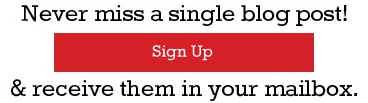Many students have not been able to handle the new pattern CAT. As expected, time management and selection of questions proved to be the biggest problems in this pattern. While VALR was not really a problem, most of the students were unable to apply the 3 Round strategy in QADI. To explain the process of test taking I attempted the New Pattern Mock CAT 1 and am sharing the process that I followed in attempting the paper.

In a paper without sectional time limits, I always start with English Usage and the Verbal Logic questions, follow it up with RC, then to Reasoning and finally to QA and DI. This ensures that I start with a strong area (Verbal Logic, vocabulary, fill in the blanks etc) and also end with my strong area (QADI), my weak area RC is hence sandwiched in between the strong areas. Also since QADI is my strongest area it is relatively less affected by fatigue that I would encounter in the last part of the paper. The time allocation for the paper is usually biased towards the section that I am stronger since it will help me get a higher overall score. For this paper too I followed a similar pattern as given below:

• Time Allocation: 80 minutes for VALR and 90 minutes for QADI
• Section Sequence: VALR followed by QADI
• VALR:
• Round1: I went from Q51-100 sequentially and attempted the questions of Verbal Logic and English Usage. While going through these questions I also took a note of the RC passages and LR sets and tried to identify which of these should be attempted. This took me about 35 minutes in which I attempted 14 out of 17 questions.
• Round 2: Now I moved to RC, in R1 I had identified that I was not comfortable with the first passage (Nietzche) and hence would not attempt it. I also identified that the last three passages (Mind reading, Modern Medicine and Ireland) were subjects that I was comfortable with and would like to attempt these three. I was not sure about the second passages (OEL Manga) and decided that I would look at it only if I had time. I attempted 9 out of 10 questions from the three passages in a little over 20 minutes.
• Round 3: As in the case of RC, in R1 I evaluated the LR sets also. I felt that the first LR set (Jungles & Animals) may not be worth attempting as it has a large amount of data. The last two LR sets (Exchange of notes and Symptoms & Diseases) appeared to be simple and were my first preference. The second LR set (Phone calls and meetings) also was data intensive but appeared to be less complicated than the first set (Animals). The LR sets of “Exchange of Notes” and “Diseases” were easy and I was able to solve them in about 11-12 minutes. I then moved to the set on “Phone calls & meetings”, I however  made mistakes and was unable solve it in the first go, I solved it in the second attempt but spent around 15-16  minutes for this data set. In the LR part hence I had an attempt of 11 questions.
• I wasted at least 5 minutes in the above LR set and if this time wastage had not happened then I would have gone back to RC and attempted one of the two remaining RC passages.
• Total attempt in VALR was 34 questions.
• Quantitative Aptitude:My sequence of attempts in this sections was:
• Round 1: I went sequentially from Q1-50 and attempted only those questions that I was sure of solving and that I felt could be attempted in 60-75 seconds. In R1 I also evaluated which questions could be considered for R2 and also which DI sets could be attempted and in which sequence. I also identified that Questions 16, 17 and 23 were not worth attempting. In R1 I attempted 13 questions in about 35 minutes.
• Round 2: I started this Round with the 5 DS questions and then moved on to the DI sets. Since I felt that the first and the last DI set would be time consuming, I attempted the remaining three that I felt were easy to understand and not calculation intensive. These 14 DI & DS questions took me about 25 minutes.
• With another 30 minutes at hand, I now shifted my focus to the QA questions that had been identified as R2 questions and attempted 6 more QA questions in approximately 20 minutes. I also wasted 2-3 minutes in solving Q1 but was unsuccessful and left it unanswered.
• Round 3: In the remaining 10 minutes I attempted the last DI set partially and a QA question that I had missed out.
• The write up below will help you understand the process of how I attempted the QADI section.

Q1. R2 – TSD – Circular Race, it is lengthy to read and looks confusing.

Q2. R1 – TSD, this can be solved by ratios. After point Karju drove at a speed that is 20% higher or 6/5 times the normal speed

• Karju should take 5/6 times the normal time for the distance CB
• Karju saves 1/6 of the normal time for the distance CB
• Time saved = 30 min (reached early by 15 min + 15 min of stoppage)
• Normal time for CB = 30 x 6 = 180 min = 3 hr
• Normal time for AB = 12 – 3 = 9 hr
• Ratio of distance AB : BC = Ratio of time AB : BC = 9 : 3 = 3 : 1

Q3. R1 – Mensuration, simple question with easy numbers on area of rectangle and circle.

We need to determine the ratio of Area of circular ground to area of rectangular field

Used a SQC technique:

Area of rectangular field = 50m x 40m = 2000 m2

• second number in the answer choice should be a factor of 2000

Only choice (a) satisfies this condition, hence this is the answer.

If you are not comfortable with this then calculate the area of the circular ground also.

Correct Answer: Choice (a) 77 : 1000

Q4. R1 – Bionomial Expansion, have done this kind of question earlier
Tr+1 = 100Cr (31/5)100-r (71/8)r

For a term to be an integer r should be a multiple of 8 and (100-r) must be multiples of, or to satisfy both the conditions r and (100 – r) must be a multiple of  (5 x 8 = 40)

• r = 0, 40, 80
• there are 3 possible values of r or 3 terms of the expansion will be integers.

Q5. R1 – PLD Used SQC technique –assumed numbers.

Let SP of X and Y be Rs 40 and 30 respectively

Take two cases of profit percentage for X and Y

Case 1: Profit percentage of X and Y are 20% and 10% respective ly

• Ratio of CP of X to Y = (40/1.2) / (30/1.1)

Case 2: Profit percentage of X and Y are 40% and 20% respective ly

• Ratio of CP of X to Y = (40/1.4) / (30/1.2)

We get a different ratio in the two cases hence the answer cannot be determined.

Correct Answer: Choice (d) Cannot be determined

Q6. R2 –Numbers. Will need to make cases and hence can be time consuming.

Q7-9. R2 DI set.

Noted on the rough sheet under DI:

Q 7–9 Bank Passbook           Calculations likely to be high            Attemptable

Q10. R1 – Averages

Average weight of the 10 students of Class B who join Class A is 5 kg more than the 10 students of Class A who joined Class B.

• The increase in to weight of Class A = 5kg x 10 = 50 kg

The increase in average weight of Class A = 0.5 kg

• The number of students in Class A =  (50 / 0.5) = 100

Q11. R2 – Geometry

Q12. R1 – Numbers, use SQC technique.

As per the answer choices a + b = 126 or 189 or neither of these. Since a and b are cubes of natural numbers, let us check the cubes of natural numbers that are less than 189.

The cubes are 1, 8, 27, 64 and 125

Now 189 = 125 + 64 and 126 = 125 + 1
Case 1: take a = 125 and b = 64, the equation in the question is satisfied, hence 189 is possible.

Case 1: take a = 125 and b = 1, the equation in the question is satisfied, hence 126 is also possible.

Correct Answer: Choice (d) Either (a) or (b)

Q13. R2 – Coordinate Geometry, likely to be time consuming

Q14. R2 – Coordinate Geometry, likely to be time consuming

Q15. R1 – Coordinate Geometry, used SQC technique
Taking θ = α = 45o , we get

sin (θ + α) = sin 90 = 1 and

a = b and c = a√2

Substituting b and c in the choices in terms of a, we find that while Choice (a) gives a value = 1, the others do not and hence Choice (b), (c) and (d) are incorrect.

Correct

Q16. R3 – Algebra, not sure of how to solve,likely to be time consuming

Q17. R3 –Numbers, I have not done this kind of question earlier and not sure of the method to be employed.

Q18-20. R2 DI set.
Noted on the rough sheet under DI:

Q 18–20 Line Chart               Simple numbers, easy calculations likely     Attemptable To be preferred over Q7-9

Q21. R3 – Numbers – Probability, not sure of how to solve,likely to be time consuming

Q22. R2 – Geometry.

Q23. R1 – Function, solved by using SQC technique.

As per the graph, for x = 0, f(x) is (+). Putting x = 0 in the choices we find that f(x) in:

Choice (a) = -2 hence incorrect

Choice (b) = 3 hence could be correct

Choice (c) = 2 hence could be correct

Choice (d) = 7 hence could be correct

As per the graph f(1) > f(0), putting x = 1 in the remaining choices we get:

Choice (b) f(1) = 4 > f(0) hence could be correct

Choice (c) f(1) = 0 < f(0) hence incorrect

Choice (d) f(1) = 5 < f(0) hence incorrect

Q24. R1 – Algebra, solved using SQC technique
x, y and z are natural numbers. We need to find the minimum possible value of the expression.

Let x = y = z = 1

• Value of the expression = 6, thus the minimum value of the expression should be ≤ 6
• Choice (b) 36 and Choice (d) 256 are incorrect

Let x = y = 1 and z = 2

• Value of the expression = 42/4 = 10.5

Thus as the value of x, y and z increases the value of the function will increase. Thus the minimum value of the expression is when  x, y and z are minimum or when x = y = z = 1

Q25. R2 – Probability – P&C. May need to make cases and hence could be time consuming.

Q26. R2 – Geometry.

Q27. R2 – Mensuration.

Q28-30. R2 DI set.

Noted on the rough sheet under DI:

Q 28–30 Pie Chart Value & Volume share  Easy calculations likely         To be attempted

Q31. R2 – Geometry.

Q32. R1 – Time & Work. Sam prepares 48 pizzas in 9 hr

• Tom prepares 60 pizzas in 13.33% less time.

In festival season both need to prepare 96 pizzas in the same amount of time by increasing their efficiency.  Thus the increase in efficiency of:

Tom: from 60 to 96 pizzas per day è efficiency to be increased by 60%

Sam: from 48 to 96 pizzas per day è efficiency to be increased by 100%

Thus ratio of increase in efficiency of Tom : Sam = 60 : 100 = 3 : 5

Correct Answer: Choice (a) 3 : 5

Q33. R2 – Mensuration.

Q34. R1 – Numbers, solved using SQC technique of checking the choices.

Decided to check 144 and 150 since these two are multiples of 6. The number of days for which Chalk will be available if the initial order is 144 = 144 + 24 + 4 + 4/6 = 172.66

Since the number of days for which chalk is required is 175 hence another 2 chalk sticks should cover us for the additional 2.33 days.

Q35. R2 – P & C

Q36. R2 – Algebra, may need to make cases.

Q37. R2 – Geometry & Trigonometry.

Q38-40. R2 DI set.
Noted on the rough sheet under DI:

Q 38–40 Table Equity shares            Calculations likely     To be attempted

Q41. R1 – Inequality, solved using SQC technique of checking the choices.
x = 0 satisfies the equation

• Choice that does not contain x = 0 should be incorrect
• Choice (a), (b) and (c) are incorrect

Correct Answer: Choice (d) x < 0

Q42. R1 – Algebra, solved by using SQC technique of taking values of x and y
Took x = 3 and y = 2 and calculated the values of LHS and RHS of the four choices, this eliminated Choice (a) and (d)
Choice (b) can be written as (xy)x > (xy)y, this will hold if both x and y are greater than 1, but not for x, y between 0 and 1, hence this is incorrect.

Correct Answer: Choice (c) xxyy > xyyx

Q43-47. R2 – Data Sufficiency

Q48-50. R2 DI set.
Noted on the rough sheet under DI:

Q 48–50 Line charts              High on calculations               Attemptable

Summary of Round 1:

• Time taken: approximately 35 minutes
• Attempted 13 questions – Q 2, 3, 4, 5, 10, 12, 15, 23, 24, 32, 34, 41 & 42.  These are, for me, the easiest QA questions in the paper and will ensure a decent score in the section.
• Identified that out of the five DI sets I would be more comfortable attempting
• Q 18-20
• Q 28-30
• Q 48-50
• Identified that Q 16, 17 and 21 should not be attempted.

Round 2:

Started R2 with the 5 DS questions

Q43-47. DS, Marked the answers of the five questions correctly.

Q38-40. DI Set Q38. Revenue generated by divestment of:

BEL = 20% of 75% of (8 cr  x 60) = 15% of 480 cr = 72 cr

BML = 25% of 60% of (3.64 cr x 15) = 15% of 55 cr = 8.25 cr
Calculations:

3.64 x 15 ≈ 3.66 x 15 = 45 + 10 = 55

15% of 55 = 5.5 + 2.75 = 8.25 cr
Total revenue = 72 + 8.25 ≈ 80 cr

Correct Answer: Choice (a) Rs 80.19 cr

Q39. Govt. owns 66.7% of 30 cr shares of BPL = 20 cr

Incremental revenue had the Govt. sold its holding at market price = (175 – 125) x 20 = 1000cr

Correct Answer: Choice (b) Rs 1000.5 cr

Q39. Decline in share price of BCL = 350 – 50 = Rs 50

• Decline in value = 50/350 = 1/7

Value of shares of Govt. = 80% of (1.515 cr x 350)

• Decline in the value of shares of Govt = 80% of (1.515 cr x 350)/7

= 80% of (1.515 cr x 50) = 1.515 x 40 ≈ 60 cr

Correct Answer: Choice (c) Rs 60.6 cr

Q28-30. DI Set

Q28. Maximum price will be for the brand which has the highest ratio of Value share to Volume share, check this ratio for the choices:

Choice (a) B = 20/10 = 2

Choice (b) D = 8/13 < 2 hence incorrect

Choice (c) C = 23/17 < 2 hence incorrect

Choice (d) F = 19/7 > 2 hence the answer

Q29. Volume sales of Brand E = 20% of the total sales

Volume sales of half of Brand B = 5%

Thus answer = 20% + 5% = 25%

Q30. Ratio of volume sales of the three types of pen

Ink Pen : Ball Point : Thin Nib = 7 :12: 1

• Share of Ink pens in the total Pen market = 7/20

Share of Chinese Ink Pen in the Ink Pen market = 15%

• Share of Chinese Ink Pens = 15% of (7/20)
• Angle subtended by Chinese Ink Pens in the Pie chart of Pens = (15% of 7/20) x 360o = 15% of (7 x 18) = 15% of 126 = 12.6 + 6.3 = 18.9o

Q18-20. DI Set

Q18. Total sales of Foto E = 26,800

Total Sales of Foto G = 39,700

• Absolute difference in sales of Foto E and Foto G = 12,900
• Monthly average of difference in sales of Foto E and Foto G = 12,900 /6 = 2150

Q19. Foto X sales in January = 3,600

Foto X sales in June = 6,600

The growth rate of sales from January to June is the same as that of June to December

• (Sales in December/Sales in June) = (Sales in June /Sales in January)
• Sales in December/6600 = 6600/3600 = 11/6
• Sales in December = 6600 x 11 / 6 = 12100

Q20. To determine the maximum growth rate, check the graph for the maximum slope and the lowest base.

Foto E from February to March increases from 3200 to 6000

• Growth rate 2800 on 3200 = 7/8 = 87.5%

At this stage I have attempted a total of 27 questions – 13 questions in R1 and in R2 I have attempted 5 DS questions and 9 questions of DI. My estimate was that the remaining 2 DI sets would be time consuming hence I moved on to the QA questions that I had not attempted earlier.

Q1. Tried solving the question but get confused regarding the position of A. Could not interpret the line “…meeting B for the first time at a point 30 m behind the starting point of A”. Wasted 2-3 minutes on this question and then left it.

Q11.  Applied SQC technique to get the answer

Drew the figure to scale, Angle EPB appears to be ≥ 60o
Cos 60o = ½ = 0.5 and Cos 90o = 0,

thus the value of the x in cos-1 x should be slightly less than 0.5
Sin 60o = √3 / 2 = 0.85 and Sin 90o = 1,

thus the value of the x in Sin-1 x should be slightly more than 0.85
Now, check the choices

Choice (a) ≈ cos-1 0.45, could be correct.

Choice (b) ≈ sin-1 0.67, incorrect

Choice (c) ≈ cos-1 0.90 incorrect

Choice (d) ≈ cos-1 0.67, incorrect

Q22. Drew a triangle ABC,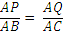, assumed P and Q to be mid points of AB and AC

• PQ is half and parallel of BC

R is a point on BC, area of triangle PQR =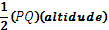Altitude of triangle PQR = perpendicular distance between PQ and BC which is will be the same irrespective of where R is on BC.

Q25. I took some time to solve this question since I had to make cases.

The number to be formed uses the digits from 1 to 8 without repetition, for this number to be a multiple of 11 the sum of its even and odd placed digits should be the same.

Digit sum of this number = 36

• Sum of digits in the even place = sum of digits in the odd place = 18

Possible combinations of the digits that add to 18 are:

(1, 4, 5, 8) and (2, 3, 6, 7)

(1, 4, 6, 7) and (2, 3, 5, 8)

(2, 4, 5, 7) and (1, 3, 6, 8)

(3, 4, 5, 6) and (1, 2, 7, 8)

When (1, 4, 5, 8) are in the even places then (2, 3, 6, 7) will be in the odd place and vice-a-versa, thus there are 2 ways of arranging the groups of the digits,

Also the digits (1, 4, 5, 8) and (2, 3, 6, 7) each can be arranged in 4! ways,

• thus the total number of arrangements =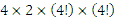Total number of ways in which 8 digits can be arranged =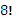Probability =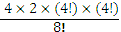= 4/35

Q33. I applied SQC technique to get the answer.

Choice (a) 6 cm is incorrect. Since the rectangle itself is of 6 cm x 12 cm so the radius of the circle should be greater than 12 cm.

In the question APQR is a 6cm x 12cm rectangle, assumed it to be a square of the same perimeter or the side of the square APQR will be 9cm.

Now the diagonal of APQR will lie on diagonal of square ABCD
Diagonal of square APQR = 9√2

Diameter of the circle = Side of square = 2r, thus radius of circle = r

Now applying Pythagoras theorem we can say,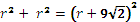or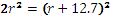Substitute choices to verify:

Choice (b) 42cm        LHS = 3528           RHS = 54.72 ≈ 3000 hence incorrect.

Choice (c) 30cm        LHS = 1800            RHS = 42.72 ≈ 1800 hence correct.

Q35. In the five digit number the even places have to be occupied by 0, 2, 4, 6 and 8

A digit can appear up to two times, thus the even places can be occupied in 5 x 5 = 25 ways
The odd digits have to occupy the three odd places and the various possibilities are:

All three different = 5 x 4 x 3 = 60 ways

Two digits are same = 5 x 1 x 4 ways = 20 ways, but these are three such possibilities, 1st & 3rd same, 1st & 5th same and 3rd & 5th same, thus the total number of arrangements = 20 x 3 = 60

Total number of ways of arranging the odd placed digits = 60 + 60 = 120
Total number of 5 digit numbers that can be formed = 25 x 120 = 3000

Q36. To find the maximum value of (p – q), p should be maximum and q should be minimum

Maximum value of p occurs when x =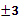, y = 2 and z = 1 thus p = 18

Minimum value of q occurs when x = -3, y = 2 and z = 1 thus q = -12

• Maximum value of (p – q) = 30

Q37. ABCD is a rectangle, hence applying Pythagoras Theorem we find that AP = 14

In triangle APB applying Sine Rule we can say: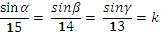• sin α = 15k,      sin β = 14k       and sin γ = 13k

As θ increase from 0o to 90o, sin θ increase from 0 to 1, since sin α > sin β > sin γ

• α > β > γ

Correct answer: Choice (d) α > β > γ

This ends my R2 and I have attempted a total of 34 questions as given below:

Round 1 – 13 questions

Round 2 – 5 DS, 9 DI and 7 more QA questions = 21 Round 3:

In the remaining 9-10 minutes I decided to check the last DI set Q48-50

Q48-50. Checked the three questions, realized that Q48 will be lengthy to solve while Q49 and Q 50 have simple calculations and decided to leave Q 48 and solve the remaining two.

Q49. Yield of wheat in 1969 =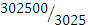= 100

Yield of what in 1965 =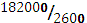Percentage increase in yield of wheat =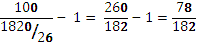> 40% but < 50%

Q50. Yield of paddy in 1970 =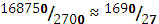Yield of what in 1971 =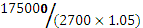Thus Percentage increase in yield of paddy =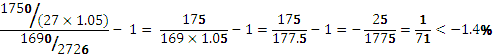Calculation – 169 x 1.05 = 169 + 5% of 169 = 169 + (16.9/2) ≈ 177.5

Correct answer: Choice (c) 1.21% less

With less than five minutes left, I went through the unattempted questions and realized that I had missed Q27, a question that I knew how to solve. Attempted the question and closed the test.

Summary of Performance

 Q. No. Question Type Attempted Not Attempted and Remarks Correct Incorrect Wasted Time 1 TSD R2 Confusing but can be solved – Revise 2 TSD R1 3 Mensuration R1 SQC: 2nd number factor of 2000 4 Bionomial R1 5 PLD R1 6 Numbers Well left, good method – Revise 7 DI  – Line Chart Well left – Tried afterwards, can be solved but is the most time consuming DI set in the paper – Revise 8 9 10 Averages R1 11 Trigonometry R2 SQC: found the range of answer 12 Algebra R1 SQC: checked choices 13 Coordinate Geometry Solveable, but okay to leave. Revise 14 Coordinate Geometry Solveable, but okay to leave. Revise 15 Trigonometry R1 SQC: took θ = α = 45o 16 Algebra Well left, not to be attempted future 17 Numbers – HCF Solveable, but okay to leave. Revise 18 DI R2 19 R2 20 R2 21 Numbers – Prob. Solveable, but okay to leave. Revise 22 Geometry R2 SQC: took P & Q  as midpoints 23 Functions/Graph R1 SQC: took x= 0 & 1 24 Algebra R1 SQC: took values of x, y & z 25 Probability – P&C R2 Revise 26 Geometry Missed in R2, To be attempted in future 27 Mensuration R3 Almost missed this question, need to be careful. 28 DI – Pie Chart R2 29 R2 30 R2 31 Geometry Should have attempted, bad miss. 32 T & W R1 33 Mensuration R2 Could have been solved without SQC. Revise 34 Numbers R1 SQC: Checked choices 35 Probability – P&C R2 Revise 36 Algebra R2 Should have attempted in R1 37 Trigonometry R2 Sine rule – Revise 38 DI – Table R2 39 R2 40 R2 41 Inequality R1 SQC: checked choices 42 Algebra R1 SQC: took values 43 DataSufficiency R2 44 R2 45 R2 46 R2 47 R2 48 DI – MultipleLine Charts Could have been answered 49 R3 50 R3 Total 13+21+3 1 14

Wishing you the very best.
GP